﻿ Basic Function Models You Must Know

# BASIC FUNCTION MODELS YOU MUST KNOW

• PRACTICE (online exercises and printable worksheets)

There are many functions that you should have on your fingertips, in the following sense:

• given the function, you should immediately be able to sketch its graph (indicating all important features)
• given the graph, you should immediately recognize the (probable) function
The purpose of this lesson is to give you practice with these basic models, which will be used freely throughout the rest of the course.

Some of these functions (e.g., the trigonometric functions) are not studied until later in the course,
but you can begin to familiarize yourself with the graphs now.

First review:   Basic Models You Must Know
The current lesson builds on this prior information.

Most graphs are shown in the same window, from $\,-5\,$ to $\,5\,$ on the x-axis, and from $\,-5\,$ to $\,5\,$ on the y-axis.
You may use the navigation arrows (bottom right on each graph) to zoom or move around.
Drag the point on each graph to see coordinates.
The scales on the x-axis and y-axis are identical. That is, a distance of $\,1\,$ on the x-axis is the same as a distance of $\,1\,$ on the y-axis.the identity function $f(x) = x$constant functions $f(x) = k\,$, $\,k\in\Bbb R$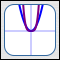the squaring function (and higher even powers) $f(x) = x^2\,$, $f(x) = x^{\text{even}}$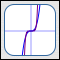the cubing function (and higher odd powers) $f(x) = x^3\,$, $f(x) = x^{\text{odd}}$the reciprocal function $\displaystyle f(x) = \frac 1x\,$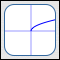the square root function $f(x) = \sqrt{x}\,$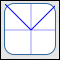the absolute value function $f(x) = |x|$the natural exponential function $\displaystyle f(x) = {\text{e}}^x$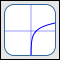the natural logarithm function $f(x) = \ln(x)\,$the sine function $f(x) = \sin(x)$the cosine function $\displaystyle f(x) = \cos(x)$the tangent function $f(x) = \tan(x)\,$

the identity function
$f(x) = x$
• The identity function takes an input, does nothing to it, and gives back the same thing as its output.
That is, the identity function preserves the identity of its input—hence its name is appropriate.
• $\,\text{dom}(f) = \mathbb{R}$
$\,\text{ran}(f) = \mathbb{R}$
• end behavior:
as $\,x \rightarrow \infty\,$, $\,y\rightarrow\infty$
as $\,x\rightarrow -\infty\,$, $\,y\rightarrow -\infty$
• $f$ increases everywhere

constant functions
$f(x) = k$
(here, $k = 3$)
• A constant function takes an input, and returns a specific real number—a constant.
In the graph at left, the function always returns the number $\,3\,$.
Constant functions are extremely boring—they ignore the input, and always return the same output.
• $\,\text{dom}(f) = \mathbb{R}$
• The range of a constant function is a set containing only one number.
For the constant function shown:
$\text{ran}(f) = \{3\}$
• important feature:
Every constant function graphs as a horizontal line.

the squaring function
(and higher even powers)
$f(x) = x^2$ (blue)
$f(x) = x^4$ (purple)

detail on behavior near zero
• The squaring function takes an input and squares it (i.e., multiplies it by itself).
• All functions of the form $\,f(x) = x^n\,$ where $\,n\in\{2,4,6,\ldots\,\}\,$
(that is, $\,f(x) = x^{\text{even}}\,$) have the same general shape, domain, range, and end behavior.
• $\,\text{dom}(f) = \mathbb{R}$
$\,\text{ran}(f) = [0,\infty)$
• end behavior:
as $\,x \rightarrow \infty\,$, $\,y\rightarrow\infty$
as $\,x\rightarrow -\infty\,$, $\,y\rightarrow \infty$
• $f\,$ decreases on $\,(-\infty,0]\,$ and increases on $\,[0,\infty)$
• important features:
• There is a horizontal tangent line at the point $\,(0,0)\,$.
• All graphs pass through the points $(0,0)$, $(1,1)$ and $(-1,1)$.
• As the even exponent gets bigger, the graph gets flatter (closer to zero) on the interval $(-1,1)$.
• As the even exponent gets bigger, the graph gets steeper (closer to vertical)
to the right of $\,1\,$ and to the left of $\,-1\,$

the cubing function
(and higher odd powers)
$f(x) = x^3$ (blue)
$f(x) = x^5$ (purple)

detail on behavior near zero
• The cubing function takes an input and cubes it ($\,x^3 = x\cdot x\cdot x\,$).
• All functions of the form $\,f(x) = x^n\,$ where $\,n\in\{3,5,7,\ldots\,\}\,$
(that is, $\,f(x) = x^{\text{odd}}\,$) have the same general shape, domain, range, and end behavior.
• $\,\text{dom}(f) = \mathbb{R}$
$\,\text{ran}(f) = \mathbb{R}$
• end behavior:
as $\,x \rightarrow \infty\,$, $\,y\rightarrow\infty$
as $\,x\rightarrow -\infty\,$, $\,y\rightarrow -\infty$
• $f\,$ increases everywhere
• important features:
• There is a horizontal tangent line at the point $\,(0,0)\,$.
• All graphs pass through the points $(0,0)$, $(1,1)$ and $(-1,-1)$.
• As the odd exponent gets bigger, the graph gets flatter (closer to zero) on the interval $(-1,1)$.
• As the odd exponent gets bigger, the graph gets steeper (closer to vertical)
to the right of $\,1\,$ and to the left of $\,-1\,$

the reciprocal function
$\displaystyle f(x) = \frac 1x$
• The reciprocal function takes the reciprocal (i.e., multiplicative inverse) of an input.
• $\,\text{dom}(f) = (-\infty,0) \cup (0,\infty)$
$\,\text{ran}(f) = (-\infty,0) \cup (0,\infty)$
• end behavior:
as $\,x \rightarrow \infty\,$, $\,y\rightarrow 0$
as $\,x\rightarrow -\infty\,$, $\,y\rightarrow 0$
• behavior near zero:
as $\,x \rightarrow 0^{+}\,$, $y\rightarrow\infty$
as $\,x \rightarrow 0^{-}\,$, $y\rightarrow -\infty$
• $f$ decreases on $\,(-\infty,0)\,$
$f$ decreases on $\,(0,\infty)\,$

the square root function
$f(x) = \sqrt{x}$
• The square root function takes the square root of an input.
• $\,\text{dom}(f) = [0,\infty)$
$\,\text{ran}(f) = [0,\infty)$
• end behavior:
as $\,x \rightarrow \infty\,$, $\,y\rightarrow\infty$
• $f$ increases everywhere on its domain
• important feature:
There is a vertical tangent line at the point $\,(0,0)\,$.

the absolute value function
$f(x) = |x|$
• The absolute value function takes the absolute value of an input.
If an input is positive or zero, then it is not changed.
If an input is negative, then the function returns its opposite.
Consequently, the absolute value always returns a nonnegative number.
• $\,\text{dom}(f) = \Bbb R$
$\,\text{ran}(f) = [0,\infty)$
• end behavior:
as $\,x \rightarrow \infty\,$, $\,y\rightarrow\infty$
as $\,x \rightarrow -\infty\,$, $\,y\rightarrow\infty$
• $f$ decreases on $\,(-\infty,0]$ and increases on $\,[0,\infty)$
• important feature:
There is a ‘kink’ (i.e., a sharp change in direction) at the point $\,(0,0)\,$.
That is, if you imagine yourself walking along the graph, going from left to right,
then at the instant you are passing through the point $\,(0,0)\,$, you will abruptly change direction.

the natural exponential function
$f(x) = {\text{e}}^x$
or
$f(x) = \exp(x)$

other bases:
$y = 2^x$
$y = 3^x$
$y = (\frac{1}{2})^x$

detail
There is an entire family of exponential functions;
they are of the form $\,y = b^x\,$, where $\,b\,$ is a positive number, not equal to $\,1\,$.
For example, $\ y = 2^x\$ and $\ y = (\frac{1}{2}){}^x\$ are exponential functions.
Thus, exponential functions have a constant base; the variable is in the exponent.

Of all the exponential functions, $\,f(x) = {\text{e}}^x\,$ (base $\,\text{e}\,$) is singled out as most important,
due to the simplicity of a calculus property it possesses:
the slope of the tangent line at the point $\,(x,{\text{e}}^x)\,$ is $\,m = {\text{e}}^x\,$;
that is, the $y$-value of the point gives the slope of the tangent line
If $x = 100\,$, the the slope of the tangent line there is $\,100\,$,
so the $y$-values are increasing $\,100\,$ times faster than the inputs!
(Exponential functions with other bases have a similar, but not-so-simple property.)
Exponential functions get big FAST!
$f(x) = {\text{e}}^x\,$ is given the special name ‘the natural exponential function’ and is also denoted by $\,f(x) = \exp(x)\,$.

Recall that $\,\text{e}\,$ is defined as the number that $\,(1 + \frac 1n)^n\,$ approaches as $n\rightarrow\infty\,$; $\,\text{e}\,$ is irrational; $\,\text{e}\approx 2.71828\,$.

If you hear the phrase ‘the exponential function’ (meaning only one) then the function being referred to is the natural exponential function.

Properties that ALL exponential functions share:
• $\,\text{domain} = \Bbb R$
$\,\text{range} = (0,\infty)$ (outputs are strictly positive; graph lies strictly above the $x$-axis)
• $y$-intercept:   $\,(0,1)\,$
• Each input has exactly one output (the graph passes the vertical line test),
and each output has exactly one input (the graph passes the horizontal line test).
Thus, exponential functions are one-to-one functions, and hence have inverses.
(Their inverses are the logarithmic functions!)
Properties dependent on the base:
 base greater than $\,1\,$ base between $\,0\,$ and $\,1\,$ end behavior as $\,x \rightarrow \infty\,$, $\,y\rightarrow \infty$ as $\,x \rightarrow -\infty\,$, $\,y\rightarrow 0$ as $\,x \rightarrow \infty\,$, $\,y\rightarrow 0$ as $\,x \rightarrow -\infty\,$, $\,y\rightarrow \infty$ increasing/decreasing increases everywhere decreases everywhere

the natural logarithm function
$f(x) = \ln(x)$

other bases:
$y = \log_2(x)$
$y = \log_3(x)$
$y = \log_{1/2}(x)$

detail
There is an entire family of logarithmic functions;
they are of the form $\,y = \log_b(x)\,$, where $\,b\,$ is a positive number, not equal to $\,1\,$.
For example, $\ y = \log_2(x)\$ and $\ y = \log_{1/2}(x)\$ are logarithmic functions.

When the base is the irrational number $\,\text{e}\,$ the function is given a special name, $\,f(x) = \ln(x) := \log_{\text{e}}(x)\,$.
Of all the logarithmic functions, $\,f(x) = \ln(x)\,$ is singled out as most important,
due to the simplicity of a calculus property it possesses:
the slope of the tangent line at the point $\,(x,\ln x)\,$ is $\,m = \frac 1x\,$;
that is, the reciprocal of the $x$-value of the point
gives the slope of the tangent line
If $x = 100\,$, the the slope of the tangent line there is only $\,\frac{1}{100}\,$,
so the $y$-values are increasing only $\,\frac{1}{100}\,$ times as fast as the inputs!
(Logarithmic functions with other bases have a similar, but not-so-simple property.)
Logarithmic functions get big, but they get big VERY SLOWLY!
$f(x) = \ln(x)\,$ is given the special name ‘the natural logarithm function’.

Recall that $\,\text{e}\,$ is defined as the number that $\,(1 + \frac 1n)^n\,$ approaches as $n\rightarrow\infty\,$; $\,\text{e}\,$ is irrational; $\,\text{e}\approx 2.71828\,$.

Some disciplines use $\,\log(x)\,$ (no indicated base) to mean the natural logarithm;
some disciplines use $\,\log(x)\,$ to mean the common logarithm (base ten).
You'll need to check notation.

Properties that ALL logarithmic functions share:
• $\,\text{domain} = (0,\infty)$ (logs only know how to act on positive numbers)
$\,\text{range} = \Bbb R$
• $x$-intercept:   $\,(1,0)\,$
• Each input has exactly one output (the graph passes the vertical line test),
and each output has exactly one input (the graph passes the horizontal line test).
Thus, logarithmic functions are one-to-one functions, and hence have inverses.
(Their inverses are the exponential functions!)
Properties dependent on the base:
 base greater than $\,1\,$ base between $\,0\,$ and $\,1\,$ end behavior as $\,x \rightarrow \infty\,$, $\,y\rightarrow \infty$ as $\,x \rightarrow \infty\,$, $\,y\rightarrow -\infty$ behavior near zero as $\,x \rightarrow 0^+\,$, $\,y\rightarrow -\infty$ as $\,x \rightarrow 0^+\,$, $\,y\rightarrow \infty$ increasing/decreasing increases on its domain decreases on its domain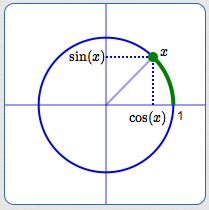the sine function
$f(x) = \sin(x)$

the cosine function
$f(x) = \cos(x)$

The sine function gives the $y$-values of points on the unit circle.
The cosine function gives the $x$-values of points on the unit circle.
(See the discussion below to understand how to associate real numbers, $\,x\,$, with points on the unit circle.)

Both sine and cosine share the following properties:
• $\,\text{domain} = \Bbb R$
$\,\text{range} = [-1,1]$   (points on the unit circle have $x$-values and $y$-values between $\,-1\,$ and $\,1\,$)
• important feature:
periodic, with period $\,2\pi\,$

## AssociatingReal NumbersWith Points onthe Unit Circle

Start with a circle centered at the origin with radius $\,1\,$, as shown below.
Sine and cosine are both defined using this unit circle;
the other trigonometric functions are defined in terms of sine and cosine.

We must associate the real numbers with points on this unit circle.
To do this, think of the real number line as an infinite string:
• black dot at $\,x=0\,$
• positive numbers colored green
• negative numbers colored red
Pick up this piece of string and wrap it around the circle as follows:
• put the black dot at the point $(1,0)$ on the circle
• wrap the positive numbers counterclockwise (start up)
• wrap the negative numbers clockwise (start down)
Of course, the green and red will overlap, as they continue to wrap around and around the circle. (Only a short piece of green and red ‘string’ are shown here!)

In this way:
• every real number ends up at a unique point on the unit circle
• every point on the unit circle is associated with infinitely many real numbers, each $\,2\pi\,$ units apart
Remember that the circumference of the circle is:
$2\pi r = 2\pi(1) = 2\pi$
So, when you think about sine and cosine (and the other trigonometric functions) acting on a real number $\,x\,$, you want to think of $\,x\,$ in this way!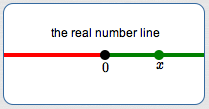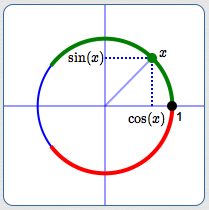the tangent function
$f(x) = \tan(x)$
The tangent function is defined as: $$\tan(x) := \frac{\sin(x)}{\cos(x)}$$
• the domain excludes all numbers of the form $\,\displaystyle \frac{\pi}2 + k\pi\,$, where $\,k\,$ is an integer
$\,\text{ran}(f) = \Bbb R$
• important feature:
periodic, with period $\,\pi\,$
Master the ideas from this section